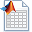• Matlab中求程序运行时间三种方法
千次阅读
2021-07-07 10:49:13

### 方法一（使用次数最多）：

计算程序运的行时间，可以在这段程序的第一句前添加语句“tic”，在该端程序后添加语句“t=toc”即可，那么返回值t即为该段程序所耗时间。

tic
%代码段
toc
%disp(['运行时间: ',num2str(toc)]);

num2str作用是将数字转字符串，disp是显示结果。时间单位：‘s’(秒)

### 方法二：

计算程序运的行时间，clock加etime函数;

t1=clock;
%代码段
t2=clock;
etime(t2,t1);

其中clock命令是获取系统的时间矢量，而etime函数则是计算两个时间矢量之间的差并以秒单位形式表示。clock作为时间矢量包含了年月日时分秒六个参数。

### 方法三：

计算程序运的行时间，cputime函数;它是使用cpu的主频计算的。

t1=cputime
%代码段
t2=cputime-t1

更多相关内容
• matlab中，为了验证比较两个算法直接的效率，我们常常需要计算某段程序的运行时间，而常用的也就是三种方法
• ## Matlab运行时间过长

千次阅读 2021-04-18 06:01:04
我是个新手，不太了解matlab，从昨天开始，代码开始运行，已经运行了超过一天了，不知道是不是自己的代码有问题。%网咯连接间隔时间分布(无APs)function y=intctime(data)[mm,nn]=size(data);intcdata=zeros(mm,3);...

我是个新手，不太了解matlab，从昨天开始，代码开始运行，已经运行了超过一天了，不知道是不是自己的代码有问题。%网咯连接间隔时间分布(无APs)

function y=intctime(data)

[mm,nn]=size(data);

intcdata=zeros(mm,3);

flag=0;

mark=inf;

t=1;

for  i=1:mm-1

k=data(i,1);

l=data(i,2);

for j=i+1:mm

if ((k==data(j,1))&&(l==data(j,2)))||((l==data(j,1))&&(k==data(j,2)))

if (data(j,3)>data(i,4))&&((data(j,3)-data(i,4))

intcdata(t,:)=[k l data(j,3)-data(i,4)];

mark=data(j,3)-data(i,4);

flag=1;

else if data(i,3)>data(j,4)&&((data(i,3)-data(j,4))

intcdata(t,:)=[k l data(i,3)-data(j,4)];

mark=data(i,3)-data(j,4);flag=1;

end

end

end

if flag==1

flag=0;

t=t+1;

mark=inf;

end

end

[Y I]= sort(intcdata(:,3));

csize=max(size(intcdata));

y=[0 0];

for i=1:csize

y(i,:)=[Y(i) (csize-i+1)/csize];

y(i,:)

end

end

这是函数，我调用的语句是temp=intctime(rmdata{3})，数据见附录

我想没有一种方法能节省运行的时间。优化一下算法2014-1-28 14:44 上传

点击文件名下载附件

1.1 MB, 下载次数: 2

里面有rmdata数据

展开全文• 此函数返回自当前 MATLAB 进程启动以来的正常运行时间（经过的时间）。 返回格式为：MATLAB up DD days, HH:MM:SS
• 这样做是有意阻止第三方拨款的（例如，我可以接受要求代码的人，我想我也可以接受出于任何原因不想提出该要求的人，并且而是决定花一些时间来构建它的临时功能版本-我想对您来说很荣誉，但是为什么呢？ circledrop.m...
• 计算运行时间的办法 Elapsed time is 0.757994 seconds. tic和toc是用来记录matlab命令执行的时间 tic用来保存当前时间，而后使用toc来记录程序完成时间。 两者往往结合使用，用法如下： tic operations toc 显示...

## 计算运行时间的办法

Elapsed time is 0.757994 seconds.

tic和toc是用来记录matlab命令执行的时间
tic用来保存当前时间，而后使用toc来记录程序完成时间。
两者往往结合使用，用法如下：
tic
operations
toc
显示时间单位： 秒

**

先把矩阵的大小确定再给矩阵的赋值的方法比边赋值边改变矩阵维数的方法更节省时间。

**

Matlab里面的计时函数：Matlab的计时函数主要有tic,toc,cputime和etime等，计时函数可以定量的计算完成制定程序所消耗的时间资源，因为可以作为比较程序优劣的一个重要标准。

cputime函数

cputime函数返回从调用该函数起所用的总的Cpu时间，单位以秒计算。

格式如：

t=cputime;

任意表达式或者程序

e=cputime-t;

etime函数

e=etime(t2,t1)命令返回向量t1和t2之间的时间段，t1和t2必须含有由clock函数返回的6个元素，即[Year Month Day Hour Minute Second]。

clc,clear;
x=rand(2048,1);
t=clock;
x=x+1;
etime(clock,t);

展开全文• % 时间窗口 params.load=10;% 载荷 params.cityNumber=7; % 城市数量 params.v=50;%平均速度 params.a1=2.5;%装卸效率 params.c1=200;% 运输固定成本 params.c2=1; % 运输变动成本 params.R=R; % 路阻系数 params...

这三段代码有问题吗？红字部分

1、function flag=test(params,city_range)

%判断个体是否满足要求

demand=params.demand ;

cityNumber=params.cityNumber;

v=params.v;

a1=params.a1;

time_window=params.time_window;

dd=params.dd;

k=1;

q=0;

d=dd(city_range(1),8);

A(city_range(1))=d/v;

q=demand(city_range(1),6);

for j=2:cityNumber

d=d+dd(city_range(j),city_range(j-1));

q=q+demand(city_range(j),6);

t(city_range(j),city_range(j-1))= dd(city_range(j),city_range(j-1))/v;

A(city_range(j))=t(city_range(j-1))+ t(city_range(j),city_range(j-1))+a1*demand(city_range(j));

if time_window(city_range(j))>A(city_range(j))

t(city_range(j))=time_window(city_range(j));

else

t(city_range(j))=A(city_range(j));

end

if q>qvechile|| A(city_range(j))>time_window(city_range(j))

k=k+1;

end

iffun(city_range,params,1)>=42

flag=0;

else

flag=1;

end

end

2、function city_range=Code(params)

%该函数用于初始化个体

flag=0;

cityNumber=params.cityNumber;

while flag==0

city_range=randperm(cityNumber);

flag=test(params,city_range);

end

end

3、

%%遗传算法多目标模型主程序

clc

clear

dbstop if error

params.dd=dd; % 距离矩阵

params.time_window=time_window;% 时间窗口

params.cityNumber=7;        % 城市数量

params.v=50;%平均速度

params.a1=2.5;%装卸效率

params.c1=200;% 运输固定成本

params.c2=1;        % 运输变动成本

params.R=R;        % 路阻系数

params.G=G;        % 高原反应系数

params.Wm=Wm;% 物资总量

params.p=500;% 惩罚值

params.demand=demand;

%%初始化遗传算法参数

maxgen=1;%进化代数,即迭代次数

sizepop=100;        %种群规模

pcross=0.8;        %交叉概率选择,0和1之间

pmutation=0.1;        %变异概率选择,0和1之间

individuals=struct('fitness',zeros(sizepop,1),'chrom',[]); %将种群信息定义为一个结构体

%%初始化种群计算适应度值

%初始化种群

for i=1:sizepop

%随机产生一个种群

individuals.chrom(i,:)=Code(params);

%计算适应度

individuals.fitness(i)=fun(individuals.chrom(i,:),params,1); %染色体的适应度

end

%找最好的染色体

[bestfitness, bestindex]=min(individuals.fitness);

bestchrom=individuals.chrom(bestindex,:); % 最好的染色体

avgfitness=sum(individuals.fitness)/sizepop; %染色体的平均适应度

%记录每一代进化中最好的适应度和平均适应度

trace=[avgfitness bestfitness];

%%迭代寻优

%进化开始

for i=1:maxgen %选择

individuals=Select(individuals,sizepop);

%交叉

individuals.chrom=Cross(pcross,individuals.chrom,params);

%变异

individuals.chrom=Mutation(pcross,individuals.chrom,params);

%计算适应度

for j=1:sizepop

x=individuals.chrom(j,:); %解码

individuals.fitness(j)=fun(x,params,1);

end

%找到最小和最大适应度的染色体及它们在种群中的位置

[newbestfitness,newbestindex]=min(individuals.fitness);

[worestfitness,worestindex]=max(individuals.fitness);

%代替上一次进化中最好的染色体

if bestfitness>newbestfitness

bestfitness=newbestfitness;

bestchrom=individuals.chrom(newbestindex,:);

end

individuals.chrom(worestindex,:)=bestchrom;

individuals.fitness(worestindex)=bestfitness;

avgfitness=sum(individuals.fitness)/sizepop;

trace=[trace;avgfitness bestfitness]; %记录每一代进化中最好的适应度和平均适应度

end

%进化结束

%%结果分析

[r, c]=size(trace);

plot(trace(:,2),'r-');

hold on

plot(trace(:,1))

xlim([1 length(trace)])

legend('最优适应度值','平均适应度值')

grid on

title('适应度曲线','fontsize',12);

xlabel('进化代数','fontsize',12);ylabel('适应度','fontsize',12);

disp('适应度变量');

x=bestchrom;

%窗口显示

disp([bestfitness x]);

展开全文•  %时间步长 precision=1e-9; %精度量级1 options=odeset('reltol',precision); N=20; NN=N/0.01; tic U=5 %[T1,Y1]=ode45(@uncontrol,[0:step_size:N],[0.001 0],options,U); [T2,Y2]=ode45(@control,[0:step_size...
• 使用Matlab运行程序的时候，我们经常需要知道或比较不同程序的具体运行时间，其程序代码如下：st=cputime; % 程序开始运行时的cpu时间% 程序开始……% 程序结束et=cputime-st % 计算程序运行所用的时间经常我们需要...
• matlab统计代码运行多少次文献资料 介绍 包pv（多项式值）实现评估机箱的方法一个区间内多项式范围的取值。 多项式可以有区间系数。 方法基于以下形式： 霍纳（pvhornerenc，pvhornerbzenc，pvhornerlzenc） ...
• 使用matlab进行时间序列分析，预测效果如文档所示，代码运行简单，修改方便。已做成工具箱,只需替换数据即可运行
• 可调参数值可以在 MATLAB 工作区中的仿真运行期间更改，并可用于影响当前正在进行的 Simulink 仿真运行。 非可调参数也在 MATLAB 工作区中定义，但对于单个仿真运行是固定的。 在附加的模型中，嵌入式 MATLAB
• matlab时间检测函数，比起自带的tic toc，运行时间更稳定
• 下面我们就说说MATLAB中计算程序运行时间的三种常用方法吧！ 注意：三种方法由于使用原理不一样，得到结果可能有一定的差距！ 1、tic和toc组合(使用最多的) 计算tic和toc之间那段程序之间的运行时间，它的经典...
• 本人在数学建模过程中撰写的matlab代码，完全可用，功能是利用BP神经网络对时间序列进行预测，内含matlab格式的数据，便于运行检验。
• 迭代任务的解决方案有时需要比预期更长的时间。 解决问题有两种可能，要么等待程序完成任务，要么中断计算机运行。 这两种选择都令人不快。 在第一种情况下，计算可能需要多长时间或是否进入无限循环存在相当高的不...
• 资源名：MATLAB ARIMA 模型 做时间序列分析预测 matlab源码 资源类型：matlab项目全套源码 源码说明： 全部项目源码都是经过测试校正后百分百成功运行的，如果您下载后不能运行可联系我进行指导或者更换。 适合人群...
• 我们想运行时和真实时间同步时，有两种方法： Real-time synchronization 模块 有的电脑不支持real-time这个模块（好像是这个模块需要读取电脑的硬件时钟，有的电脑不让外部软件读取，造成这个模块失效） 2. ...开发语言
• matlab 命令与程序清单 (matlab commands and functions list) A a chi2cdf 分布累计概率函数 abs 绝对值模字符的 ASCII 码值 acos 反余弦 chi2inv 分布逆累计概率函数 acosh 反双曲余弦 acot 反余切 chi2pdf 分布...
• 同样的效果不同的实现方法，运行时间和内存消耗大不相同。今天分享MATLAB运行时间分析方法及一些简单的提速技巧。
• 时序预测 | MATLAB实现LSTM时间序列未来多步预测（完整源码和数据） 数据为一维时序列数据,运行环境MATLAB2018b及以上，可以实现未来100个值的预测。
• ## MATLAB运行效率

千次阅读 2020-12-16 01:14:45
用过Matlab的人都知道，Matlab是一种解释性语言，存在计算速度慢的问题，为了提高程序的运行效率，matlab提供了多种实用工具及编码技巧。1. 循环矢量化Matlab是为矢量和矩阵操作而设计的，因此，可以通过矢量化方法...
• disp( ['运行时间: ',num2str(toc) ] ); 则输出结果为———— 运行时间: 48.0364s 2.如果计算多段程序的时间 tic num1= calculate_number(x1,y1); toc time1=toc; tic num2= calculate_number(x2,y2); toc time...
• 文章目录代码格式注意 代码 tic for i=1:100 data(i)=i end toc % disp(['运行时间: ',num2str(toc)]); ...%disp(['运行时间: ',num2str(toc)]);...1、运行同样的程序，每次运行的...2、最后一行disp(['运行时间: ',nu...
• Data的缩写）是一个用于时间序列数据管理的MATLAB软件包。 它允许从Haver，FRED和Bloomberg中检索。 包括常规的时间序列操纵功能，以及其他一些用于管理将相关序列合并为复合序列的方法。 从概念上讲，它分为三大...
• 对于Matlab用户来说，解决方案很简单：如果您没有时间重新编写例程，请在Matlab内部运行R代码。 您需要在您的机器上安装 R 的工作版本。 然后将您的 R 命令保存到一个文本文件中（通过 Word 或其他文本编辑器），......

# matlab的运行时间是什么matlab 订阅